### Home > INT3 > Chapter 10 > Lesson 10.1.5 > Problem10-71

10-71.

Match each equation with the appropriate graph. Do this without using a graphing calculator.

1. $y = \text{sin}( x + \frac { \pi } { 2 } )$

1. $y = \text{sin}\left(2x\right)$

1. $y = 2\text{sin}( \frac { x } { 2 } )$

1. $y = \text{sin}\left(x\right) - 3$

1. $y = -\text{sin}( 2 ( x - \frac { \pi } { 8 } ) )$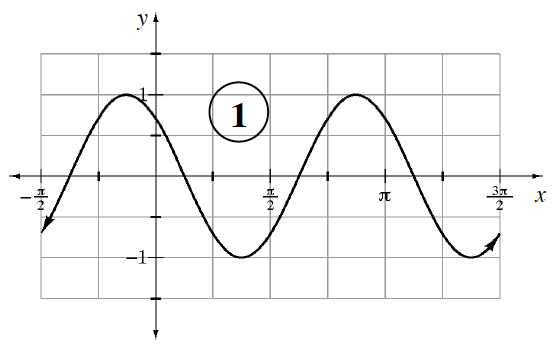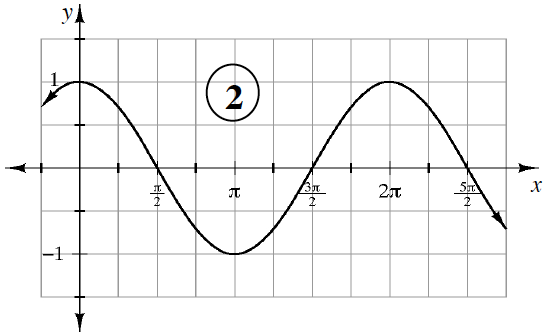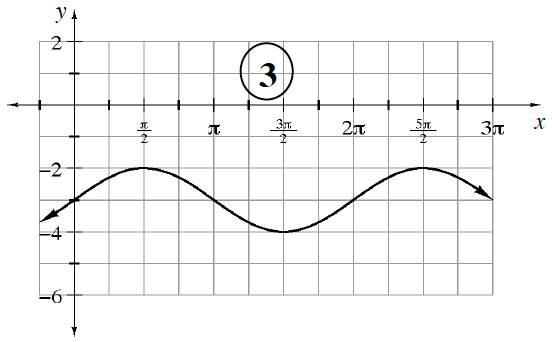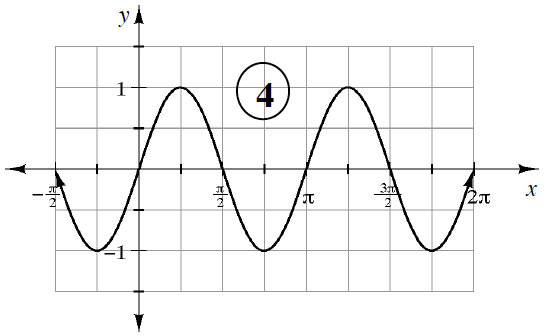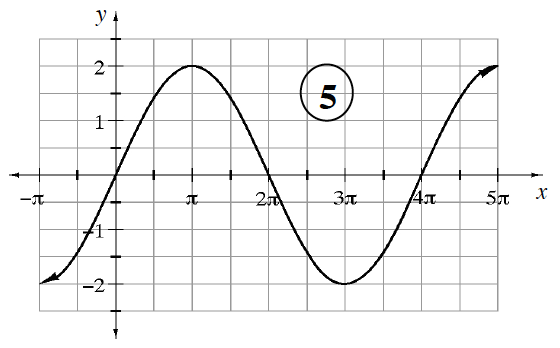(a) 2
(b) 4
(c) 5
(d) 3
(e) 1